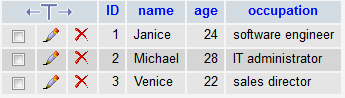﻿ How to Concatenate Column Values of a MySQL Table Using Python ﻿# How to Concatenate Column Values of a MySQL Table Using PythonIn this article, we show how to concatenate column values of a MySQL table using Python.

What is meant by this is that we can take data from different columns and put them together. Concatenating column values means that you get values from multiple columns in one MySQL statement and can combine these values to do anything. This could mean you could form a statement based on the column values. Or you could do arithmetic such as add column values together or multiply them or anything. MySQL has a built-in function Concat() that allows you to concatenate any column values together and you can do whatever you want with those values.

Let's say we have a table named Employees.

This table, Employees, has the columns, name and occupation.

With concatenation, we can combine a statement that can put all of the information across all columns together.

Thus, we can put together in a single statement, name works as this job as an occupation.

The code to concatenate columns value in MySQL is shown below.

So the above is the MySQL concatenate a column named name and a column named occupation. In between these 2 column values, we insert a string ' works as a '. Therefore, we output the person's name, then the ' works as a ' string, and then the occupation. So if one of the rows is Janice and her occupation is a software engineer, the full line will be, Janice works as a software engineer. And we've just concatenated 2 column values together.

This is the power of concatenation across columns of a MySQL table.

So, now, let's go over a real-life example.

Below, we have a table named Employees that has 3 columns: name, age, and occupation.In our code, we will concatenate all these 3 columns, so that we write that name, age years old, works as an occupation.

The full Python code for this is shown below.

So, this is all the code that is needed to concatenate the 3 columns, so that it reads the name, age, and occupation of a worker in a single statment.

So we first must import MySQLdb. Once that is imported, we gain connection to the MySQL database using the MySQLdb.connect() function. To see a full-length article on connecting to a MySQL database, see How to Connect to a MySQL Database in Python.

We then have to create a cursor for the table.

Next, we execute our function to concatenate the 3 columns using the cursor.execute() function. Inside of this function, we place in the line, "SELECT Concat(name, ', age ', age, ', works as a ', occupation) AS fulldetail FROM Employees". So the whole line of code, is, cursor.execute("SELECT Concat(name, ', age ', age, ', works as a ', occupation) AS fulldetail FROM Employees")

We then create a variable named result and set it equal to cursor.fetchall().

We then use a for loop and print out i, which represents each of the rows representing an employee.

We then close the database once we've done what we've needed to.

Running the above code gives us the following output.

So, you can see how it prints out each of the statements about each employee.

And this is how we can concatenate multiple columns of a MySQL using Python.

Related Resources